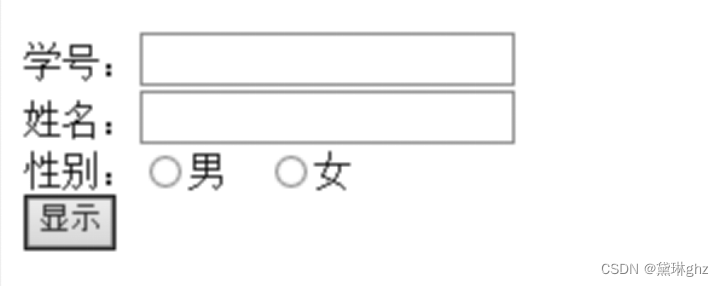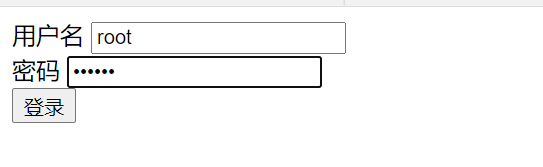# PHP学习笔记3-表单

+关注继续查看

## PHP 表单处理

PHP 超全局变量 $_GET$_POST 用于收集表单数据（form-data）

### 一个简单的 HTML 表单

<html>
<body>
<form action="welcome.php" method="post">
Name: <input type="text" name="name"><br>
E-mail: <input type="text" name="email"><br>
<input type="submit">
</form>
</body>
</html>

<html>
<body>
Welcome <?php echo $_POST["name"]; ?><br> Your email address is: <?php echo$_POST["email"]; ?>
</body>
</html>

### GET vs POST

GET 和 POST 都创建数组（例如，array( key => value, key2 => value2, key3 => value3, ...)）。此数组包含键/值对，其中的键是表单控件的名称，而值是来自用户的输入数据。
GET 和 POST 被视作 $_GET$_POST。它们是超全局变量，这意味着对它们的访问无需考虑作用域 - 无需任何特殊代码，您能够从任何函数、类或文件访问它们。
$_GET 是通过 URL 参数传递到当前脚本的变量数组。 $_POST 是通过 HTTP POST 传递到当前脚本的变量数组。

GET 可用于发送非敏感的数据。

## PHP表单验证

<form method="post" action="<?php echo htmlspecialchars($_SERVER["PHP_SELF"]);?>"> 当提交此表单时，通过 method=”post” 发送表单数据。 ### 什么是 $_SERVER["PHP_SELF"] 变量？

$_SERVER["PHP_SELF"] 是一种超全局变量，它返回当前执行脚本的文件名。 因此，$_SERVER["PHP_SELF"] 将表单数据发送到页面本身，而不是跳转到另一张页面。这样，用户就能够在表单页面获得错误提示信息。

### 什么是 htmlspecialchars() 函数？

htmlspecialchars() 函数把特殊字符转换为 HTML 实体。这意味着 < 和 > 之类的 HTML 字符会被替换为 &lt;&gt; 。这样可防止攻击者通过在表单中注入 HTML 或 JavaScript 代码（跨站点脚本攻击）对代码进行利用。

### 关于 PHP 表单安全性的重要提示

$_SERVER["PHP_SELF"] 变量能够被黑客利用！ 如果您的页面使用了 PHP_SELF，用户能够输入下划线然后执行跨站点脚本（XSS）。 提示：跨站点脚本（Cross-site scripting，XSS）是一种计算机安全漏洞类型，常见于 Web 应用程序。XSS 能够使攻击者向其他用户浏览的网页中输入客户端脚本。 假设我们的一张名为 “test_form.php” 的页面中有如下表单： <form method="post" action="<?php echo$_SERVER["PHP_SELF"];?>">

<form method="post" action="test_form.php">

http://www.example.com/test_form.php/%22%3E%3Cscript%3Ealert('hacked')%3C/script%3E

<form method="post" action="test_form.php"/><script>alert('hacked')</script>

<form method="post" action="<?php echo htmlspecialchars($_SERVER["PHP_SELF"]);?>"> htmlspecialchars() 函数把特殊字符转换为 HTML 实体。现在，如果用户试图利用 PHP_SELF 变量，会导致如下输出： <form method="post" action="test_form.php/"><script>alert('hacked')</script>"> 无法利用，没有危害！ ### 通过 PHP 验证表单数据 在用户提交该表单时，我们还要做两件事： 1）通过 PHP trim() 函数，去除用户输入数据中不必要的字符（多余的空格、制表符、换行） 2）通过 PHP stripslashes() 函数，删除用户输入数据中的反斜杠（\） 接下来我们创建一个检查函数（相比一遍遍地写代码，这样效率更好）。 我们把函数命名为 test_input()。 现在，我们能够通过 test_input() 函数检查每个 $_POST 变量，脚本是这样的：

<?php
// 定义变量并设置为空值
$name =$email = $gender =$comment = $website = ""; if ($_SERVER["REQUEST_METHOD"] == "POST") {
$name = test_input($_POST["name"]);
$email = test_input($_POST["email"]);
$website = test_input($_POST["website"]);
$comment = test_input($_POST["comment"]);
$gender = test_input($_POST["gender"]);
}
function test_input($data) {$data = trim($data);$data = stripslashes($data);$data = htmlspecialchars($data); return$data;
}
?>

if ($_SERVER["REQUEST_METHOD"] == "POST") { if (empty($_POST["name"])) {
$nameErr = "Name is required"; } else {$name = test_input($_POST["name"]); } if (empty($_POST["email"])) {
$emailErr = "Email is required"; } else {$email = test_input($_POST["email"]); } if (empty($_POST["website"])) {
$website = ""; } else {$website = test_input($_POST["website"]); } if (empty($_POST["comment"])) {
$comment = ""; } else {$comment = test_input($_POST["comment"]); } if (empty($_POST["gender"])) {
$genderErr = "Gender is required"; } else {$gender = test_input($_POST["gender"]); } } ?> ### 显示错误消息 在 HTML 表单中，我们在每个被请求字段后面增加了一点脚本。如果需要，会生成恰当的错误消息（如果用户未填写必填字段就试图提交表单）： <form method="post" action="<?php echo htmlspecialchars($_SERVER["PHP_SELF"]);?>">
Name: <input type="text" name="name">
<span class="error">* <?php echo $nameErr;?></span> <br><br> E-mail: <input type="text" name="email"> <span class="error">* <?php echo$emailErr;?></span>
<br><br>
Website:
<input type="text" name="website">
<span class="error"><?php echo $websiteErr;?></span> <br><br> <label>Comment: <textarea name="comment" rows="5" cols="40"></textarea> <br><br> Gender: <input type="radio" name="gender" value="female">Female <input type="radio" name="gender" value="male">Male <span class="error">* <?php echo$genderErr;?></span>
<br><br>
<input type="submit" name="submit" value="Submit">
</form>

## 表单验证 - 验证 E-mail 和 URL

### 验证名字

$name = test_input($_POST["name"]);
if (!preg_match("/^[a-zA-Z ]*$/",$name)) {
$websiteErr = "无效的 URL"; } ## 表单验证 - 完成表单实例 本节展示如何在用户提交表单后保留输入字段中的值。 ### 保留表单中的值 如需在用户点击提交按钮后在输入字段中显示值，我们在以下输入字段的 value 属性中增加了一小段 PHP 脚本：name、email 以及 website。在 comment 文本框字段中，我们把脚本放到了 <textarea></textarea> 之间。这些脚本输出 $name、$email、$website 和 $comment 变量的值。 然后，我们还需要显示选中了哪个单选按钮。对此，我们必须操作 checked 属性（而非单选按钮的 value 属性）： Name: <input type="text" name="name" value="<?php echo$name;?>">
E-mail: <input type="text" name="email" value="<?php echo $email;?>"> Website: <input type="text" name="website" value="<?php echo$website;?>">
Comment: <textarea name="comment" rows="5" cols="40"><?php echo $comment;?></textarea> Gender: <input type="radio" name="gender" <?php if (isset($gender) && $gender=="female") echo "checked";?> value="female">Female <input type="radio" name="gender" <?php if (isset($gender) && \$gender=="male") echo "checked";?>
value="male">MalePHP的表单完成是什么意思？底层原理是什么？
PHP的表单完成是什么意思？底层原理是什么？
42 094 059 0PHP通过类来获取登录表单的数据并显示
PHP通过类来获取登录表单的数据并显示
71 0PHP表单处理 ※
PHP表单处理 ※
118 0PHP表单
PHP表单
77 0php如何接受jquery表单序列化后的值
php如何接受jquery表单序列化后的值
82 0Ajax & PHP 边学边练 之四 表单
1295 0PHP获取表单方法
1065 0PHP 表单的提交完美示例
1179 0
JKXQJ

PHP完全自学手册文档教程946088

PHP 2017.北京 全球开发者大会——高可用的PHP